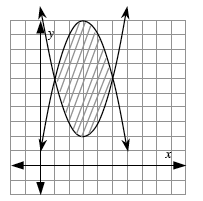### Home > PC > Chapter 4 > Lesson 4.2.3 > Problem4-102

4-102.

Sketch the following system of inequalities, estimate the area between the curves, and explain what you used to estimate the area.

$\left\{ \begin{array} { l } { y \leq - ( x - 3 ) ^ { 2 } + 10 } \\ { y \geq ( x - 3 ) ^ { 2 } + 2 } \end{array} \right.$

See graph below.
By counting squares, or using the area program we can estimate the area as $\approx21$.